# Introduction To Exponential Functions

in STEMGeeks2 months ago

Hi there. In this math guide, I introduce the topic of exponential functions. It is assumed that the reader is familiar with exponents.

For math images I use a combination of Quicklatex.com, witeboard.com and my Wacom tablet & stylus.Pixabay Image Source

## Topics

• The Exponential Function
• Determining Exponential Function From Table Of Values

## The Exponential Function

The exponential function takes an exponent input and produces an output. How the input is used to produce an output is based on the following function.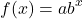where `b` is the base number, `x` is the exponent and `a` is the y-intercept. The output is `f(x)` which depends on the value of the exponent `x`.

If the function is 2 to the power of `x` you would have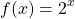From the function `f(x)` if the exponent was 1 the output would be 2 to the power of 1 which is 2. An exponent of 5 would give two to the power of 5 which is 32.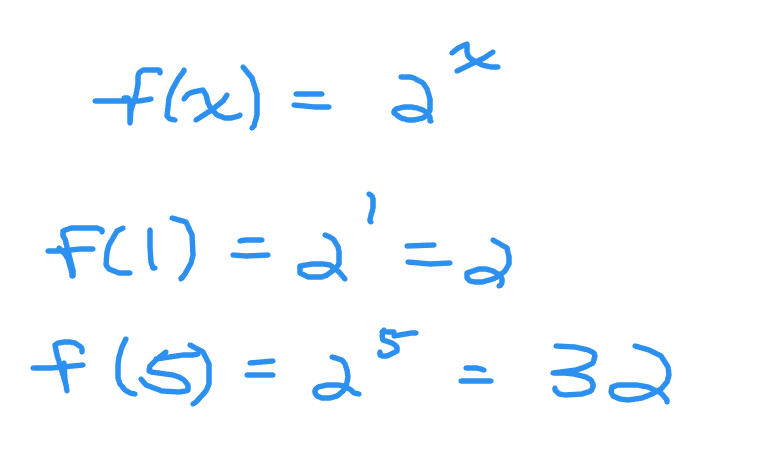If the exponent is `x = -2` you would have two to the power of negative two. This would be one divided by two to the power of two which gives `1/4`.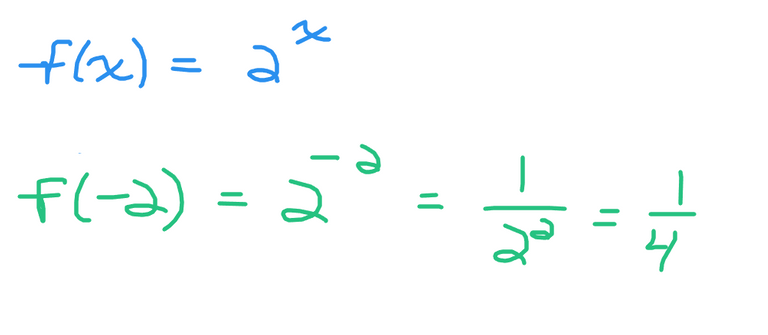We can develop a small table of values.

xf(x) = 2^x
-21/4
-11/2
01
12
24
38

## Determining Exponential Function From Table Of Values

The previous section dealt with obtain values once the exponential function is known. What if you have the opposite case? That would be using some inputs and outputs from an exponential function and finding the exact equation for it.

Example One

xy
-21/9
-11/3
01
13
29
327

The y-intercept here is 1, so a = 1.

For every time that x increases by 1, y increases by a multiple of 3 (or multiply by 3 each time x increases by 1). This multiple of 3 represents the base (b). The exponential equation for this example would be y = 3^x. Screenshot below.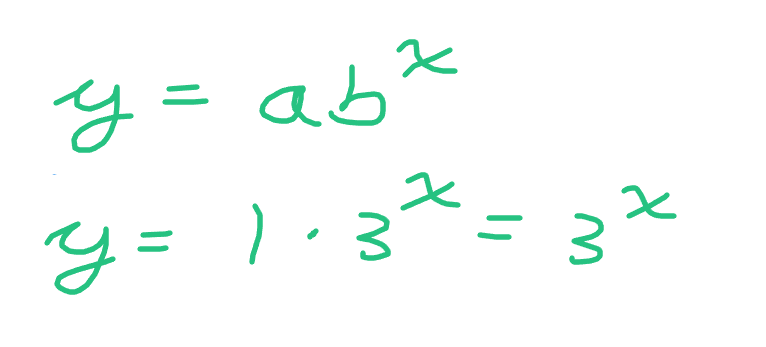Example Two

xy
-2-
-11
02
14
28
316

From the table above, the y-intercept is 2. This is the y-value when x is equal to 0. The a-value from the exponential equation here is 2.

Whenever `x` increases by 1, y increases by a multiple of 2. The base value `b` in the exponential equation is 2.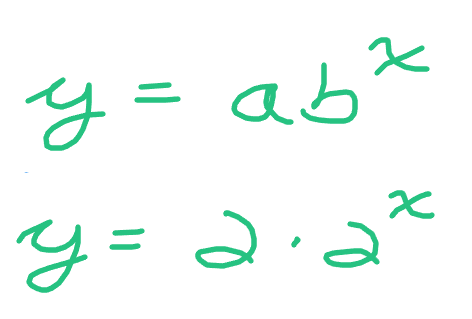Example Three

xy
-216
-14
01
11/4
21/16
31/64

In this table the y-intercept is 1. As `x` increases by 1, the y-value decreases by dividing by 4. Dividing by four is the same as multiplying by one-quarter. The base value here is one-quarter in the exponential equation.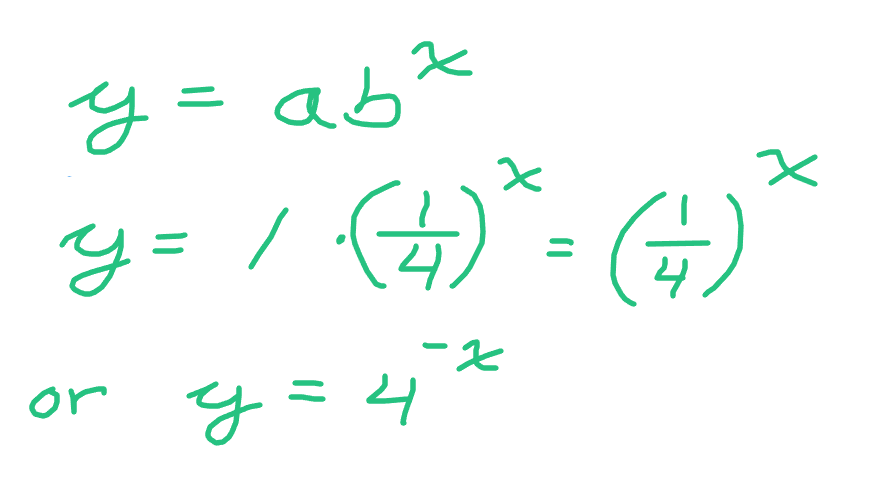Example Four - Only One Point Known

This fourth example is slightly different. Other than the y-intercept there is only one known point from the exponential equation. Algebra is used to find the pieces of the exponential function.

xy
-2-
-1-
01
1-
24
3-

From the exponential equation `y = ab^x`, substitute the known point `(2, 4)` along with `a = 1`. The value of `a` is 1 as this number one is the y-intercept. After substituting `x = 2` and `y = 4` solve for the value of `b`.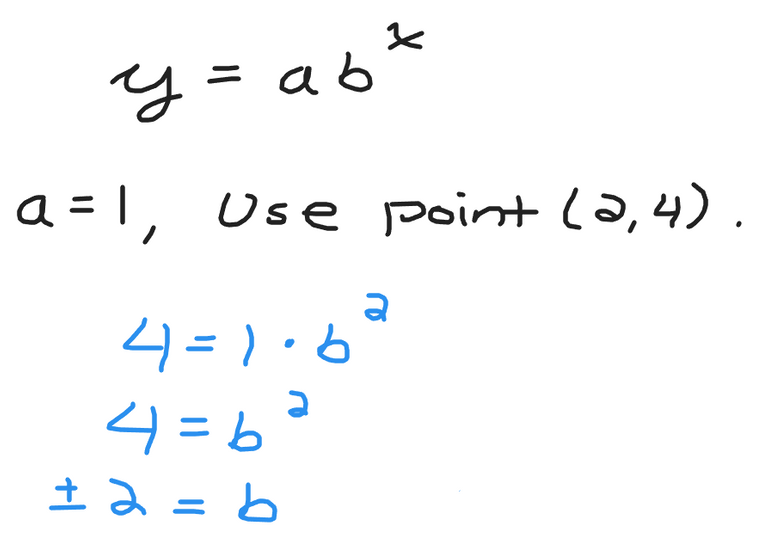The value of the base `b` can be either positive two or negative two. If the base is a negative then you do not really have an exponential function as the signs alternate between positive and negative depending on the exponent being even or odd. Take the positive two to obtain the exponential function of to the power of x.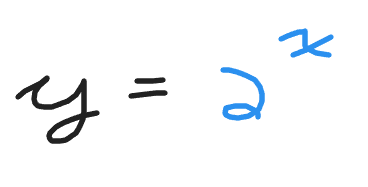Posted with STEMGeeks

Sort:

Nice introduction to the mathematics of growth. Here is some WINE, may it grow exponentially. And, yes, I know WINE is ruled by the rules of discrete mathematics and not continuous mathematics ... but it is kinda the same thing sortof.

!WINE

Congratulations, @irivers You Successfully Shared 0.100 WINEX With @dkmathstats.
You Earned 0.100 WINEX As Curation Reward.
You Utilized 1/1 Successful Calls.WINEX Current Market Price : 0.227

Swap Your Hive <=> Swap.Hive With Industry Lowest Fee (0.1%) : Click This Link

Thank you for your witness vote!
Have a !BEER on me!
To Opt-Out of my witness beer program just comment STOP below

2 months ago Reveal Comment

thats really nice
!1UP#### You have received a 1UP from @gwajnberg!

The @oneup-cartel will soon upvote you with:
@stem-curator
And they will bring !PIZZA 🍕.